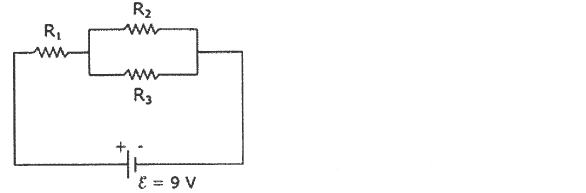# Problem: The circuit in the sketch consists of three resistors and a battery with emf ε = 9.0 V and negligible internal resistance. R1 = 2.0 Ω, R2 = 12.0 Ω, and R3 = 4.0 Ω. What is the power dissipated by each resistor? P1 = _______________ P2 = _______________ P3 = _______________

###### FREE Expert Solution
93% (187 ratings)
###### Problem Details

The circuit in the sketch consists of three resistors and a battery with emf ε = 9.0 V and negligible internal resistance. R1 = 2.0 Ω, R2 = 12.0 Ω, and R3 = 4.0 Ω.

What is the power dissipated by each resistor?

P= _______________

P= _______________

P3 = _______________Frequently Asked Questions

What scientific concept do you need to know in order to solve this problem?

Our tutors have indicated that to solve this problem you will need to apply the Solving Resistor Circuits concept. You can view video lessons to learn Solving Resistor Circuits. Or if you need more Solving Resistor Circuits practice, you can also practice Solving Resistor Circuits practice problems.

What is the difficulty of this problem?

Our tutors rated the difficulty ofThe circuit in the sketch consists of three resistors and a ...as high difficulty.

How long does this problem take to solve?

Our expert Physics tutor, Juan took 7 minutes and 32 seconds to solve this problem. You can follow their steps in the video explanation above.